# Tutorial#2.pptx

2. Nov 2022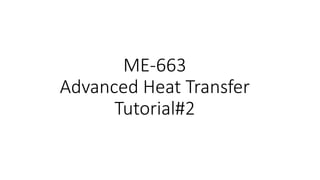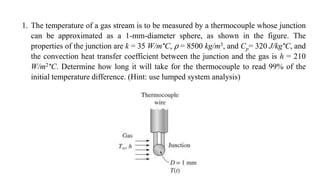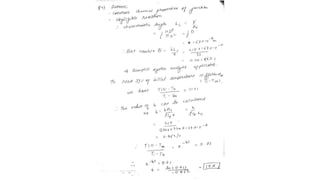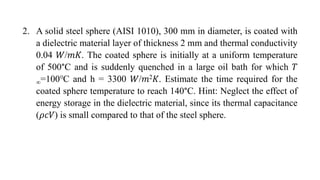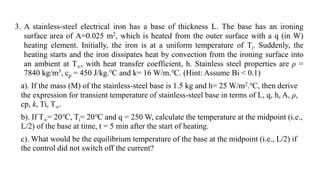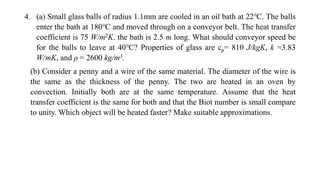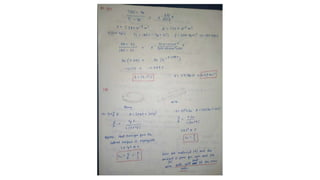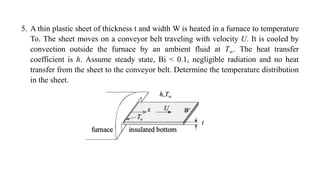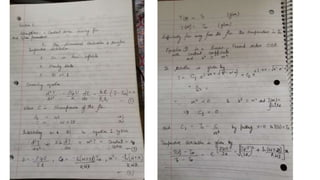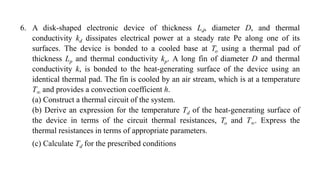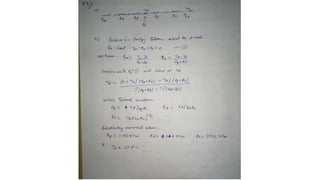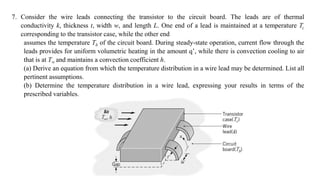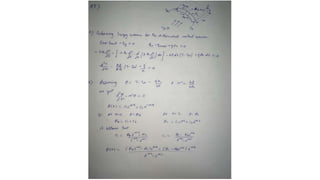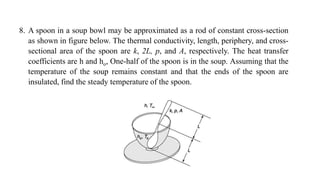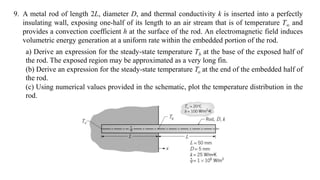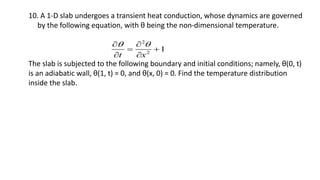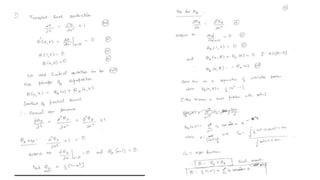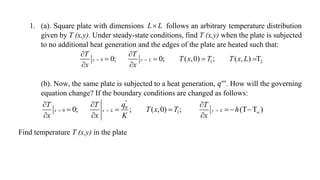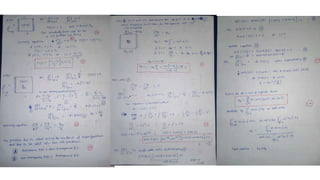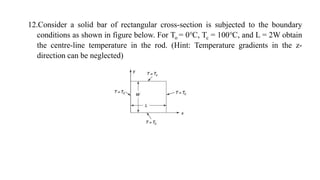1 von 20

### Tutorial#2.pptx

• 1. ME-663 Advanced Heat Transfer Tutorial#2
• 2. 1. The temperature of a gas stream is to be measured by a thermocouple whose junction can be approximated as a 1-mm-diameter sphere, as shown in the figure. The properties of the junction are k = 35 W/m°C, ⍴ = 8500 kg/m3, and Cp= 320 J/kg°C, and the convection heat transfer coefficient between the junction and the gas is h = 210 W/m2°C. Determine how long it will take for the thermocouple to read 99% of the initial temperature difference. (Hint: use lumped system analysis)
• 4. 2. A solid steel sphere (AISI 1010), 300 mm in diameter, is coated with a dielectric material layer of thickness 2 mm and thermal conductivity 0.04 𝑊/𝑚𝐾. The coated sphere is initially at a uniform temperature of 500°C and is suddenly quenched in a large oil bath for which 𝑇 ∞=100℃ and h = 3300 𝑊/𝑚2𝐾. Estimate the time required for the coated sphere temperature to reach 140°C. Hint: Neglect the effect of energy storage in the dielectric material, since its thermal capacitance (𝜌𝑐𝑉) is small compared to that of the steel sphere.
• 5. 3. A stainless-steel electrical iron has a base of thickness L. The base has an ironing surface area of A=0.025 m2, which is heated from the outer surface with a q (in W) heating element. Initially, the iron is at a uniform temperature of Ti. Suddenly, the heating starts and the iron dissipates heat by convection from the ironing surface into an ambient at T∞, with heat transfer coefficient, h. Stainless steel properties are ρ = 7840 kg/m3, cp = 450 J/kg.℃ and k= 16 W/m.℃. (Hint: Assume Bi < 0.1) a). If the mass (M) of the stainless-steel base is 1.5 kg and h= 25 W/m2.℃, then derive the expression for transient temperature of stainless-steel base in terms of L, q, h, A, ρ, cp, k, Ti, T∞. b). If T∞= 20℃, Ti= 20℃ and q = 250 W, calculate the temperature at the midpoint (i.e., L/2) of the base at time, t = 5 min after the start of heating. c). What would be the equilibrium temperature of the base at the midpoint (i.e., L/2) if the control did not switch off the current?
• 6. 4. (a) Small glass balls of radius 1.1mm are cooled in an oil bath at 22℃. The balls enter the bath at 180℃ and moved through on a conveyor belt. The heat transfer coefficient is 75 W/m2K. the bath is 2.5 m long. What should conveyor speed be for the balls to leave at 40℃? Properties of glass are cp= 810 J/kgK, k =3.83 W/mK, and ρ = 2600 kg/m3. (b) Consider a penny and a wire of the same material. The diameter of the wire is the same as the thickness of the penny. The two are heated in an oven by convection. Initially both are at the same temperature. Assume that the heat transfer coefficient is the same for both and that the Biot number is small compare to unity. Which object will be heated faster? Make suitable approximations.
• 8. 5. A thin plastic sheet of thickness t and width W is heated in a furnace to temperature To. The sheet moves on a conveyor belt traveling with velocity U. It is cooled by convection outside the furnace by an ambient fluid at T∞. The heat transfer coefficient is h. Assume steady state, Bi < 0.1, negligible radiation and no heat transfer from the sheet to the conveyor belt. Determine the temperature distribution in the sheet.
• 10. 6. A disk-shaped electronic device of thickness Ld, diameter D, and thermal conductivity kd dissipates electrical power at a steady rate Pe along one of its surfaces. The device is bonded to a cooled base at To using a thermal pad of thickness Lp and thermal conductivity kp. A long fin of diameter D and thermal conductivity k, is bonded to the heat-generating surface of the device using an identical thermal pad. The fin is cooled by an air stream, which is at a temperature T∞ and provides a convection coefficient h. (a) Construct a thermal circuit of the system. (b) Derive an expression for the temperature Td of the heat-generating surface of the device in terms of the circuit thermal resistances, To and T∞. Express the thermal resistances in terms of appropriate parameters. (c) Calculate Td for the prescribed conditions
• 12. 7. Consider the wire leads connecting the transistor to the circuit board. The leads are of thermal conductivity k, thickness t, width w, and length L. One end of a lead is maintained at a temperature Tc corresponding to the transistor case, while the other end assumes the temperature Tb of the circuit board. During steady-state operation, current flow through the leads provides for uniform volumetric heating in the amount q’, while there is convection cooling to air that is at T∞ and maintains a convection coefficient h. (a) Derive an equation from which the temperature distribution in a wire lead may be determined. List all pertinent assumptions. (b) Determine the temperature distribution in a wire lead, expressing your results in terms of the prescribed variables.
• 14. 8. A spoon in a soup bowl may be approximated as a rod of constant cross-section as shown in figure below. The thermal conductivity, length, periphery, and cross- sectional area of the spoon are k, 2L, p, and A, respectively. The heat transfer coefficients are h and ho, One-half of the spoon is in the soup. Assuming that the temperature of the soup remains constant and that the ends of the spoon are insulated, find the steady temperature of the spoon.
• 15. 9. A metal rod of length 2L, diameter D, and thermal conductivity k is inserted into a perfectly insulating wall, exposing one-half of its length to an air stream that is of temperature T∞ and provides a convection coefficient h at the surface of the rod. An electromagnetic field induces volumetric energy generation at a uniform rate within the embedded portion of the rod. a) Derive an expression for the steady-state temperature Tb at the base of the exposed half of the rod. The exposed region may be approximated as a very long fin. (b) Derive an expression for the steady-state temperature To at the end of the embedded half of the rod. (c) Using numerical values provided in the schematic, plot the temperature distribution in the rod.
• 16. 10. A 1-D slab undergoes a transient heat conduction, whose dynamics are governed by the following equation, with θ being the non-dimensional temperature. The slab is subjected to the following boundary and initial conditions; namely, θ(0, t) is an adiabatic wall, θ(1, t) = 0, and θ(x, 0) = 0. Find the temperature distribution inside the slab. 2 2 1 t x        
• 18. 1. (a). Square plate with dimensions L L  follows an arbitrary temperature distribution given by T (x,y). Under steady-state conditions, find T (x,y) when the plate is subjected to no additional heat generation and the edges of the plate are heated such that: 0 1 2 0; 0; ( ,0) ; ( , ) T x x L T T T x T T x L x x           (b). Now, the same plate is subjected to a heat generation, 𝑞’’’. How will the governing equation change? If the boundary conditions are changed as follows: '' 0 0 1 0; ; ( ,0) ; (T T ) x x L y L q T T T T x T h x x K x                Find temperature T (x,y) in the plate
• 20. 12.Consider a solid bar of rectangular cross-section is subjected to the boundary conditions as shown in figure below. For To = 0℃, Tc = 100℃, and L = 2W obtain the centre-line temperature in the rod. (Hint: Temperature gradients in the z- direction can be neglected)